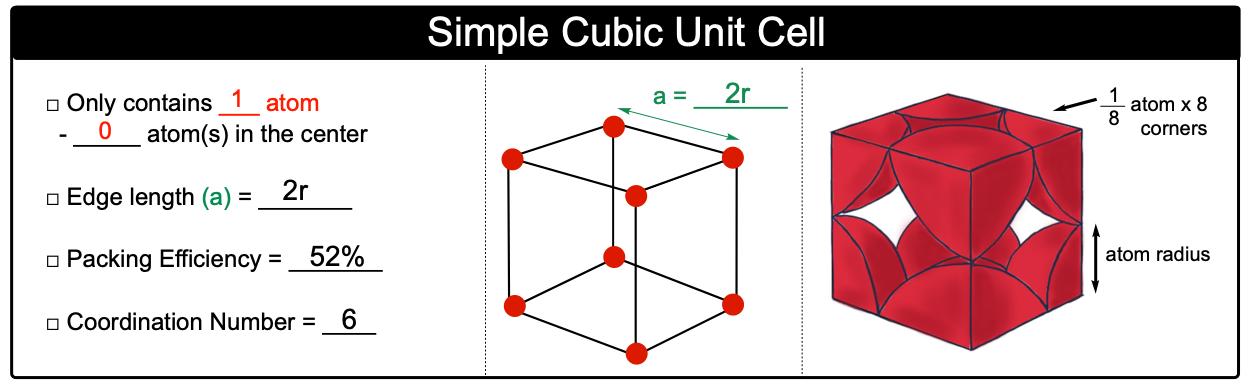Clutch Prep is now a part of Pearson
Ch.11 - Liquids, Solids & Intermolecular ForcesWorksheetSee all chapters

# Simple Cubic Unit Cell

See all sections
Sections
Molecular Polarity
Intermolecular Forces
Intermolecular Forces and Physical Properties
Clausius-Clapeyron Equation
Phase Diagrams
Heating and Cooling Curves
Atomic, Ionic, and Molecular Solids
Crystalline Solids
Simple Cubic Unit Cell
Body Centered Cubic Unit Cell
Face Centered Cubic Unit Cell

Simple Cubic Unit Cell is the least complex cubic unit cell and contains only 1 atom.

###### Simple Cubic Unit Cell

Concept #1: Simple (Primitive) Cubic Unit CellExample #1: Calculate volume in cm3 of a simple cubic unit cell if its composed of atoms with radius of 2.5 Å.

Practice: Polonium crystallizes with a primitive cubic structure. It has a density of 9.4 g/cm3, a radius of 167 pm, and a molar mass of 209 g/mol. Calculate the number of atoms in one mole of Polonium.